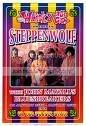-=+=- -=+=- -=+=- -=+=- -=+=- -=+=- -=+=- -=+=- -=+=- -=+=- -=+=- -=+=- -=+=- -=+=- -=+=- -=+=- -=+=- -=+=- -=+=- -=+=- -=+=- -=+=- -=+=- -=+=- -=+=- -=+=- -=+=- -=+=- -=+=- -=+=- (c) WidthPadding Industries 1987 0|743|0 -=+=- -=+=- -=+=- -=+=- -=+=- -=+=- -=+=- -=+=- -=+=- -=+=- -=+=- -=+=- -=+=- -=+=- -=+=- -=+=- -=+=- -=+=- -=+=- -=+=- -=+=- -=+=- -=+=- -=+=- -=+=- -=+=- -=+=- -=+=- -=+=- -=+=-
SoCoder -> Blogs Home -> Blogs

magicmanCreated : 30 April 2007
Edited : 30 April 2007

### Art Stuff

A little somthing for

Heres a few bits of randomness that I have made, they make for good desktops.Wednesday, 02 May 2007, 07:25
flying_cuccoPsychedelic! especially the second-to-last. Can you do higher res ones?
Wednesday, 02 May 2007, 09:08
mike_gNice, I like the third from last one bestWednesday, 02 May 2007, 18:58
magicmanyeah, i could probably do higher res, those are actually 800,600 but they've been scaled. if you right click and hit view image you can see the big version.Wednesday, 02 May 2007, 22:30
power mouseyyeah!!

psychedelic and acid too...

like Steppenwolfand Jefferson Starship